# Section 3.5. Passing a Value and Passing a Reference

[Page 118 (continued)]

### 3.5. Passing a Value and Passing a Reference

The effect of passing arguments to a method differs depending on whether you are passing a value of primitive type (such as 5 or TRue) or a value of reference type (such as "Hello" or game1). When an argument of primitive type is passed to a method, a copy of the argument is passed to the formal parameter. For example, consider the PrimitiveCall class shown in Figure 3.7. Note that we have an int variable k, which initially stores the value 5, and a method myMethod(), which takes an int parameter n. In this case, when we invoke myMethod(k), k's value (5) is copied into n and stored there during the method.

##### Figure 3.7. Passing a primitive value to a method. (This item is displayed on page 119 in the print version)

 `public class PrimitiveCall { public static void myMethod(int n) { System.out.println("myMethod: n= " + n); n = 100; System.out.println("myMethod: n= " + n); } // myMethod() public static void main(String argv[]) { int k = 5; System.out.println("main: k= " + k); myMethod(k); System.out.println("main: k= " + k); } // main() } // PrimitiveCall class `

Passing a primitive value

One implication of passing a copy of a primitive value to a method is that the original value of k in main() cannot be altered from inside the method. Thus, the output generated by PrimitiveCall would be

`main: k= 5 myMethod: n= 5 myMethod: n= 100 main: k= 5 `

[Page 119]

Note that in main(), k's value is printed both before and after myMethod() is called, but its value is unaffected even though n's value is changed within the method. This is because myMethod() contains just a copy of k's value, not k itself. Any changes to the copy within myMethod() leave k unaltered (see Fig. 3.8).

##### Figure 3.8. Tracing the state of variables k and n in PrimitiveCall: (a) Just before calling myMethod(k) in main. (b) Just before executing the body of myMethod(). (c) Just after executing the body of myMethod(). (d) After flow of control returns to main().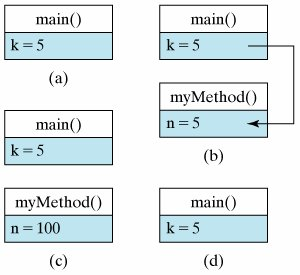Java Language Rule: Passing a Primitive Value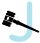When a value of a primitive type, like boolean or int, is passed to a method, a copy of the value is passed. That's why its original value remains unchanged outside the method even if the copy is changed inside the method.

In contrast to this, when an argument of a reference type is passed to a method, a copy of the reference to the object itself is assigned to the parameter. For example, in the case of a String parameter or a OneRowNim parameter, the method would be given a reference to the objectthat is, the address of the object. The object itself is not passed, because it would be too inefficient to copy the entire object with all its data and methods. However, because the object's reference gives the object's location in memory, the method will have access to the object and can make changes to the original object from within the method.

For example, consider the ReferenceCall class (Fig. 3.9). In this case, myMethod() takes a parameter g of type OneRowNim. Because a OneRowNim instance is an object, g is a reference variable. So when myMethod(game) is invoked in main(), a reference to game is passed to myMethod(). Note that in myMethod(), we use takeSticks(3) to change the number of sticks of g from 10 to 7, and this change persists even after the method returns control to main(). The reason is that during the method's execution, both game and g refer to the exact same object (see Fig. 3.10). The output generated by ReferenceCall would be

[Page 120]
##### Figure 3.9. Passing a reference value to a method.

 `public class ReferenceCall { public static void myMethod(OneRowNim g) { System.out.print("myMethod: Number of sticks: "); System.out.println(g.getSticks()); g.takeSticks(3); System.out.print("myMethod: Number of sticks: "); System.out.println(g.getSticks()); } // myMethod() public static void main(String argv[]) { OneRowNim game = new OneRowNim(10); System.out.print("main: Number of sticks: "); System.out.println(game.getSticks()); myMethod(game); System.out.print("main: Number of sticks: "); System.out.println(game.getSticks()); // main() } // ReferenceCall class `

##### Figure 3.10. Tracing the state of OneRowNim object in ReferenceCall: (a) Just before calling myMethod(game). (b) Just before executing the body of myMethod(). (c) Just after executing the body of myMethod(). (d) After flow of control returns to main().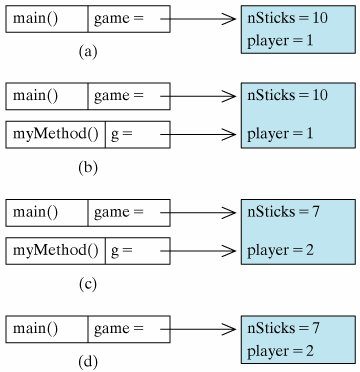`main: Number of sticks: 10 myMethod: Number of sticks: 10 myMethod: Number of sticks: 7 main: Number of sticks: 7 `

[Page 121]

This illustrates that when passing a reference variable to a method, it is possible for the method to change the state of the object associated with the reference variable. In subsequent chapters we will see ways to make use of this feature of reference parameters.

Java Language Rule: Passing a ReferenceWhen a reference to an object is passed to a method, any changes made to the object from within the method will persist when the method is finished executing.

Debugging Tip: Side Effects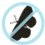An unintended change to an object is called a side effect. In designing methods, care should be taken to ensure that the method does not produce unwanted side effects in objects passed as reference parameters.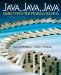Java, Java, Java, Object-Oriented Problem Solving (3rd Edition)
ISBN: 0131474340
EAN: 2147483647
Year: 2005
Pages: 275

Similar book on Amazon